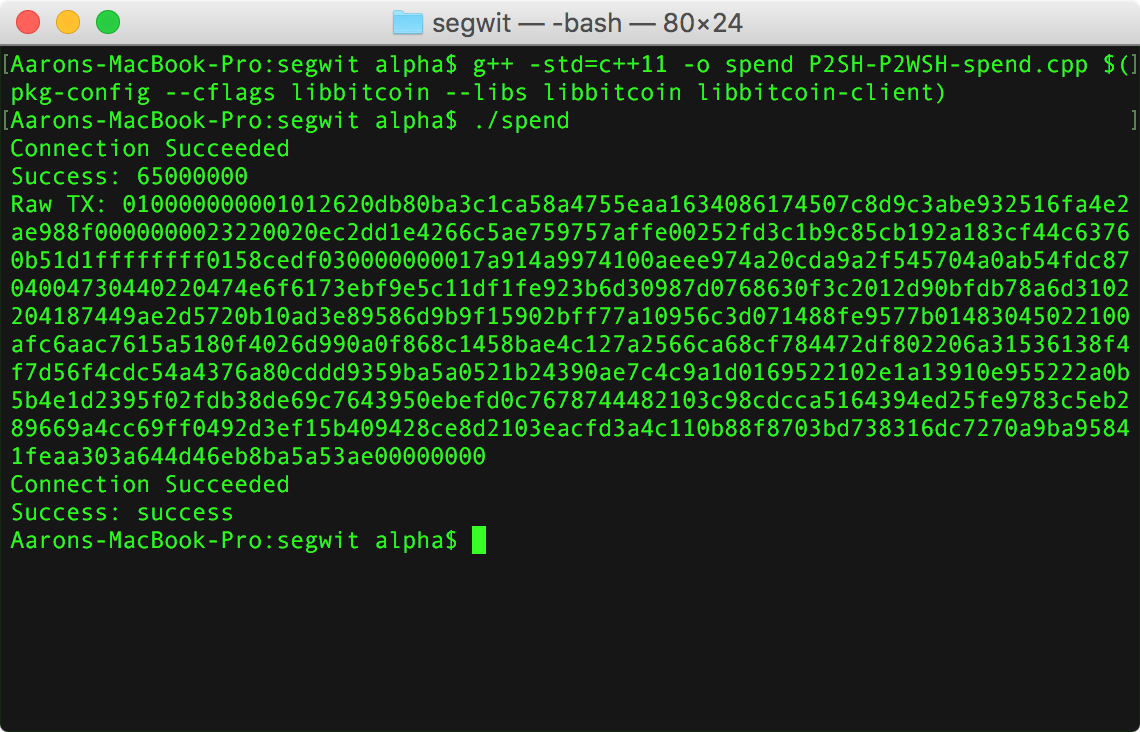# Libbitcoin: Spend a Non-Native Segwit Multisig OutputAfter you’ve constructed your P2SH-P2WSH address your going to want to be able to spend the outputs via segregated witness.

In order to follow along with this tutorial, first go to a faucet and send some tesnet coins to your multisig address.

The program we are going to write is going to use the libbitcoin-client functions previously described in transaction tutorials for getUTXOs and broadcastTX

```
#include <bitcoin/bitcoin.hpp>
#include <bitcoin/client.hpp>
#include <string.h>

using namespace bc;
using namespace bc::wallet;
using namespace bc::machine;
using namespace bc::chain;

{
client::connection_type connection = {};
connection.retries = 3;
connection.timeout_seconds = 8;
connection.server = config::endpoint("tcp://testnet1.libbitcoin.net:19091");
client::obelisk_client client(connection);

points_value val1;
static const auto on_done = [&val1](const points_value& vals) {

std::cout << "Success: " << vals.value() << std::endl;
val1 = vals;

};

static const auto on_error = [](const code& ec) {

std::cout << "Error Code: " << ec.message() << std::endl;

};

if(!client.connect(connection))
{
std::cout << "Fail" << std::endl;
} else {
std::cout << "Connection Succeeded" << std::endl;
}

client.wait();

//return allPoints;
return val1;

}
{
client::connection_type connection = {};
connection.retries = 3;
connection.timeout_seconds = 8;
connection.server = config::endpoint("tcp://testnet3.libbitcoin.net:19091");
client::obelisk_client client(connection);

if(!client.connect(connection))
{
std::cout << "Fail" << std::endl;
} else {
std::cout << "Connection Succeeded" << std::endl;
}

static const auto on_done = [](const code& ec) {

std::cout << "Success: " << ec.message() << std::endl;

};

static const auto on_error2 = [](const code& ec) {

std::cout << "Error Code: " << ec.message() << std::endl;

};

client.wait();
}

```

After those we are going to use the helper functions we defined in the create address tutorial to build the witness script and redeem script.

```
script getWitnessScript(ec_public key1, ec_public key2, ec_public key3) {
//make key list
point_list keys {key1.point(), key2.point(), key3.point()};
//create 2/3 multisig script
script multisig = script::to_pay_multisig_pattern(2u, keys);
return multisig;
}

script getRedeemScript(ec_public key1, ec_public key2, ec_public key3) {
//create 2/3 multisig script
script multisig = getWitnessScript(key1, key2, key3);

//sha256 the script
data_chunk multisig_hash = to_chunk(sha256_hash(multisig.to_data(0)));

//redeem script
operation::list redeemscript_ops {operation(opcode(0)), operation(multisig_hash)};
script redeem_script = script(redeemscript_ops);

return redeem_script;
}

```

After those, we are going to write a function to get an ec_private object from a private key string.

```ec_private stringToPrivate(std::string stringEC){
ec_secret secret;
decode_base16(secret, stringEC);
return ec_private(secret, ec_private::testnet);
}
```

Now we can start to build our transaction in the main funciton. to start we will define three private keys, corresponding to the 3 public keys we generated for the multisig.

Then we get our multisig address by passing the 3 public keys to our getRedeemScript method inside of our payment address constructor.

```
int main() {

ec_private key2 = stringToPrivate("3edef3e2d2152156e6816c06e65f64baff4fc0831cf25ae86df016a5cb651747");

```

Now, we can build our transaction object and output for our reciever.

```	transaction tx;
tx.set_version(1u);
//make output
uint64_t amount;
btc_to_satoshi(amount, "0.64999");
tx.outputs().push_back(output(amount, outScript));

```

Once we have defined where our coins are going we can get our UTXOs and start building our input. For this we are going to need to save our UTXOs amount in order to properly sign the tx.

```
//make input
uint64_t previous_amount = UTXOs.points.value();

input workingInput = input();
workingInput.set_previous_output(output_point(UTXOs.points));
workingInput.set_sequence(max_input_sequence);
tx.inputs().push_back(workingInput);

```

Now, before we sign the tx we are going to need to use the witness script as the script code, so we create a script object using the getWitnessScript function.

```//witness script
script witness_script = getWitnessScript(key1.to_public(), key2.to_public(), key3.to_public());
//
```

Then we can sign the tx, just like a P2SH-P2WKH output.

```	endorsement sig1;
script().create_endorsement(sig1, key1.secret(), witness_script, tx, tx.inputs().previous_output().index(), sighash_algorithm::all, script_version::zero, previous_amount);
```

But we need to do it again because we need signatures from at least 2 keys.

```	endorsement sig2;
script().create_endorsement(sig2, key2.secret(), witness_script, tx, tx.inputs().previous_output().index(), sighash_algorithm::all, script_version::zero, previous_amount);
```

Now that we have both signatures, we need to create the input script, which is simply a push of the redeem script data.

```	script redeem_script = getRedeemScript(key1.to_public(), key2.to_public(), key3.to_public());
script inputScript = script(to_chunk(redeem_script.to_data(1)), 0);
tx.inputs().set_script(inputScript);
```

Finally we can create the witness by creating a stack of 0 (an empty datachunk because of the script multisig bug), the two signatures and the witness script data.
we can then set this as the witness.

```	data_chunk zero;
data_stack witness_data {zero, sig1, sig2, witness_script.to_data(0)};
tx.inputs().set_witness(witness(witness_data));
```

Once we have the full transaction, we can print it to the console and broadcast it to the network.

```	std::cout << "Raw TX: " << encode_base16(tx.to_data(1, 1)) << std::endl;
```	//build